Smartick is a fun way to learn math!Oct10

# Singapore Bars Applied to Fractions

In today’s post, we are going to learn how to apply Singapore bars to problems with fractions.

As we know, we can be asked for various amounts in problems with fractions….so let’s look at a few examples so it is very clear to us! Mind you, fractional numbers have become easy for us so they are not the focus of this post but rather the point of this post is to learn how to use the bars to solve fractions.

There are 24 animals on a farm. Two-thirds of them are cows. How many cows are there?Let’s put all the facts in our bar: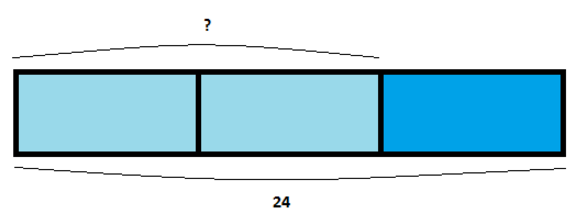Then, since we see that three units are equal to 24 animals, we can first calculate how much one unit is so that we can later multiply to find out how many animals two units are equal to. That is to say:

• 3 units = 24
• 1 unit = 24 / 3 = 8
• 2 units = 2 x 8 = 16

There are 16 cows on the farm.

Now we have a farm with 36 animals, in which two-thirds are cows. How many animals are not cows?

We draw our Singapore bar with the facts: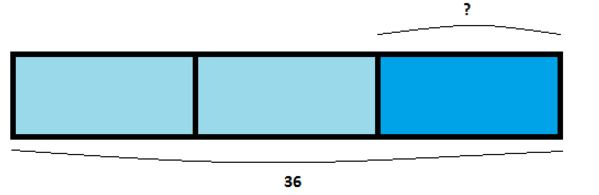This is very similar to the previous problem! The difference is that here the part that interests us is different, one third. So all we have to find out is how much one unit is worth, that is to say, divide by 3.

• 3 units = 36
• 1 unit = 36 / 3 = 12

So there are 12 animals on the farm that are not cows.

Let’s move on to the next type of problem.

I have prepared a bouquet of flowers to give to my mother. Two-thirds of the flowers are violets and the rest are daisies. If there are 16 violets in the bouquet, how many flowers in total are in the bouquet?We show this with a Singapore bar like this: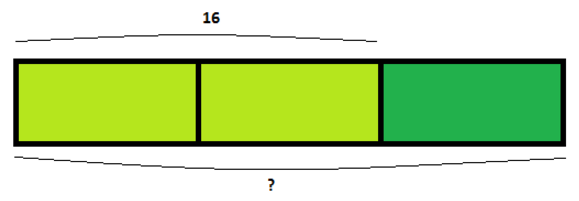The key is to do the same thing as in the previous problems: first, we calculate how much one of the parts is and then we find how much the other part that we want is, in this case, the three parts:

• 2 units = 16
• 1 unit = 16 / 2 = 8
• 3 units = 3 x 8 = 24

The bouquet has 24 flowers.

My sister wants to copy the gift, so she also has bought a bouquet of daisies and violets for my mother. If two-thirds of her bouquet are daisies and she has 20 daisies, how many violets does she have?

Let’s draw the bar: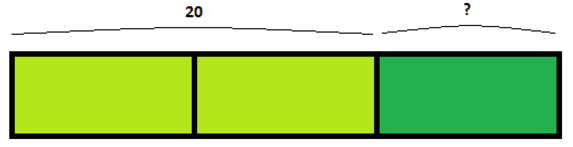It is easy to see that the daisies represent two units. So then, how much are 3 units? We find how much one unit is and then multiply by 3:

• 2 units = 20
• 1 unit = 20 / 2 = 10

The bouquet has 10 violets.

Let’s look at the last problems, which are a little more complicated but much easier with the Singapore bars!

At my camp, there are a lot of kids. I know that there are 75 girls and also that there are three fifths as many boys as girls. How many boys are there in the camp?This case is different from the previous problems because we are going to need two Singapore bars: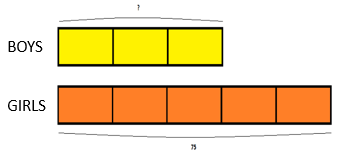Since we know how much 5 parts is, we can reduce this to one part and then find out how much 3 parts is worth:

• 5 units = 75
• 1 unit = 75 / 5 = 15
• 3 units = 15 x 3 = 45

In other words, there are 45 boys in the camp.

If they ask for the other amount it is also very easy to find:

At my camp, there are 15 boys, which represents three fifths the amount of girls. How many girls are there in the camp?

We draw the two bars: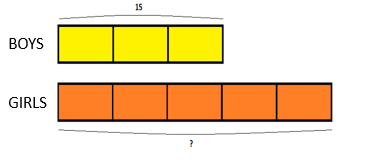This example is very similar to the previous one. We have to see how much one part is and then we can find the five parts.

• 3 units = 15
• 1 units = 15 / 3 = 5
• 5 units = 5 x 5=  25

So there are 25 girls at the camp.

Another quantity that they can ask us to find is the sum. And with this problem, we will finish this post, although there are many more examples!

My father has bought oranges and strawberries to make smoothies for my birthday party. He bought 50 strawberries and three fifths this amount of oranges. How many fruits has he bought in total?Once again, we need to use two bars: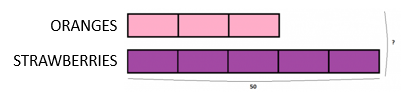Like in the previous examples, we find how much one unit is worth and then we multiply, but this time we multiply by ALL the units, that is to say, 5 + 3 = 8.

• 5 units = 50
• 1 units = 50 / 5=  10
• 8 units = 10 x 8 = 80

He has bought 80 fruits in total.

And if they give us the other amount, it is equally easy to find!

Since more friends are coming to the party, my mom went shopping and bought 45 oranges, once again, three fifths the amount of strawberries that she bought. How much fruit has she bought this time?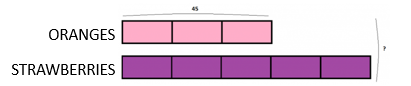The same as before, we know how much three parts is: 45 oranges. So we reduce down to one unit and then multiply by all the units.

• 3 units = 45
• 1 units = 45 / 3=  15
• 8 units = 15 x 8 = 120

So my mother bought 120 pieces of fruits in total, 45 oranges and 75 strawberries.

That’s it! Can you suggest more examples of applying Singapore bars to fractions? There are more! In Smartick we use this strategy so that you feel more comfortable and learn much more quickly, so register to challenge yourself and prove how much you have learned today.